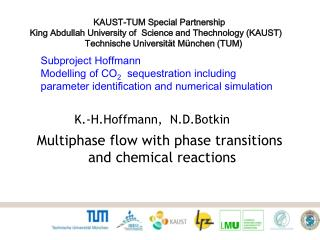DownloadDownload PresentationSubproject Hoffmann Modelling of CO 2 sequestration including

# Subproject Hoffmann Modelling of CO 2 sequestration including

Télécharger la présentation## Subproject Hoffmann Modelling of CO 2 sequestration including

- - - - - - - - - - - - - - - - - - - - - - - - - - - E N D - - - - - - - - - - - - - - - - - - - - - - - - - - -
##### Presentation Transcript

1. KAUST-TUM Special Partnership King Abdullah University of Science and Thechnology (KAUST) Technische Universität München (TUM) Subproject Hoffmann Modelling of CO2 sequestrationincluding parameteridentificationandnumericalsimulation K.-H.Hoffmann, N.D.Botkin Multiphase flow with phase transitions and chemical reactions

2. An example of CO2 sequestration An artists rendering of the Utsira CO2 injection (from the thesis of J. M. Nordbotten) Platform Gas is being produced from the Sleipner field and separated from CO2. The last component is being injected into the Utsira formation. Utsira formation CO2 injection well Pumping wells Sleipner cas field Norway

3. Specific of multiphase flows rock is the porosity rock (fl. vol./ global vol.) g saturation of a-th phase (phase vol./ fl. vol.) a b density of a-th phase A pore with three fluid phases physical density of a-th phase vel. and pres. of a-th phase 1. Different velocities and pressures of phases, capillary pressures. 2. Mass and impulse conservations are formulated for each phase, but there are no substitutive relations that couple and . 3. The saturations are unknown variables, but there are no direct equations for them.

4. Capillary pressures: hydrophobic hydrophilic wetting angle q q oil oil water water imbibition A realistic example of the capillary pressure curve drainage

5. Consider phases. components in Let be the concentration of -th component in a-th phase. is the fugacity function of a-th phase is the density of the free Gibbs energy of a-th phase , is the indicator function of the set • Phase transitions Conventional phase equilibrium relations: , , . Account for kinetics and unstable behavior of the phases in critical states: , , , is fixed is a double/multi well shallow function that is responsible for phase transitions of unstable CO2 phases, Note that the new relation transforms into the conventional fugacity relations at the stedy-state limit.

6. Chemical reactions and source/sink terms is the reaction rate of the component in the a phase: , is the injection/production rate of the a phase. The last term describes a bore hole. Roughly speaking this is the delta-function. Nevertheless there is a special technique to handle it on a very coarse grid. Peaceman law (1973), well index

7. Phase velocities: abandonment of Darcy's law Compare: Dynamics of multiphase media. R.I. Nigmatulin. Hemispere Pub.Corp. New York 1991. , , are the density, velocity, pressure, and deviatoric part of the stress tensor of a-th phase, respectively, averaging of the divergence of the stress tensor over micro motions of the a phase accounting for stresses on phase interfaces. describes the impulse transfer from the b-th to a-th phase due to the friction, in the absence of exotic interactions between phases. describes the mass transfer rate due to the b￫ a phase change, the barycentric velocity simulates the impulse transfer from the rock to the a-th phase.

8. Relation to Darcy's law is very large because of a large contact area between the rock and the medium. the relaxation time Thus, the velocity arrives at a stationary regime very quickly, and satisfies a stationary equation which resembles Darcy’s law. Proposition (S.Torquato, Random heterogeneous materials. Springer 2002) Darcy’s permeabilityform-factor micro constantDarcy’s permeability Identification: the relaxation time is equal to micro

9. Let be the number of the current time step, known state at , and the right-hand-side of the global velocity equation at but without the pressure term. , , Summation f all velocity equations over the phases yields a global equation for the barycentric velocity and a nominal pressure , . are the barycentric velocity, global density, and global deviatoric stress, respectively, , is a nominal pressure, say the pressure of a selected phase: then , where are known capillary pressures, , is responsible for chemical reactions and injections.

10. . and • Algorithm 1. Compute an auxiliary velocity from the first equation of 2. Apply the divergence operator to the second equation of step1 to obtain: . 3. Use theglobal mass conservation equation to compute: , and to obtain the following nonlinear elliptic equation: , where . • Compare: • O. M. Belotserkovsky. Numerical modeling in mechanics of continua. Moscow 1994. (Temam, 1969), (Fortin, 1971). Incompressible N-S equations.

11. 4. Compute from step 3. Then compute from the second equation of step 1. 5. Compute and solve the impulse conservation equation for . 6. Solve the mass conservation equation for , find from the relation: . 7. Repeat steps 1-7 so that under the condition:

12. Simulations (CO2)heavy , Water (middle), (CO2)light 1. Three phases: 2. Weak compressibility: 3. Absence of the capillary pressures (worth case)! 4. Phase transitions between (CO2)heavyand (CO2)light 5. Creation of (CO2)light

13. Two dimensional simulations inlet outlet Three saturations Without gravitation and blocks

14. Without gravitation but with obstacles

15. gravity With gravitation and blocks. Gravitation is directed against Y axes

16. gravity More detailed demonstration of the phase transition for the light phase

17. Rectangular region gravity With gravitation but without blocks

18. gravity With gravitation and phase creation

19. Three dimensional simulations. The light phase gravity With gravitation and blocks. Gravitation is directed against Z axes

20. The heavy phase gravity

21. The middle phase gravity

22. Another shape The light phase gravity

23. The heavy phase gravity

24. The middle phase gravity